Physics - Coulomb's law - [Static Electricity]

Coulomb's law - [Static Electricity]

Problem:

The electrostatic force between two point sized stationary electric charges is directly proportional to the product of the charges and inversely proportional to square of the distance between them and acts along the line joining them.

Explanation :

Let Q1 and Q2 be the two charges seprated by a distance 'd'. Then the electrostatic force 'F' between them is given by :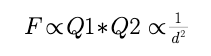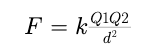In S.I system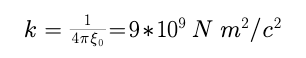where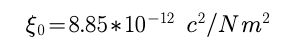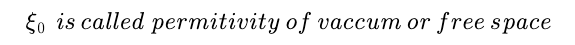Coulomb's law hold good for point charges at rest only.

Post By : Ashish Maurya 17 May, 2020 331 views Physics